# LMoFit | Advanced L-Moment Fitting of Distributions

#### 2020-11-24

Citation to this package:

Zaghloul M, Papalexiou S, Elshorbagy A (2020). LMoFit: Advanced L-Moment Fitting of Distributions. R package version 0.1.6.

# 1 Introduction

In the practice of frequency analysis, some probability distributions are abandoned due to the absence of a convenient way of handling them. Burr Type-III (BrIII), Burr Type-XII (BrXII), and Generalized Gamma (GG) distributions are examples of such abandoned distributions. Most past studies involving frequency analysis ignored the unconventional distributions regardless of their remarkable advantages. For example, Zaghloul et al. (2020) reveals the importance of using BrIII and BrXII distributions instead of the commonly used Generalized Extreme Value (gev) distribution for flood frequency analysis across Canada. Also, Papalexiou and Koutsoyiannis (2016) recommended the use of BrXII and GG distributions for describing daily precipitation across the globe.

Various conventional and unconventional distribution are covered by LMoFit, which is the first package that facilitated the use of some unconventional distributions that are:

1. Burr Type-III (BrIII)
2. Burr Type-XII (BrXII)
3. Generalized Gamma (GG)

While the commonly used conventional distributions are masked from the ‘lmom’ package by Hosking, J.R.M. (2019). These conventional distributions are:

1. Normal (nor)
2. Log-Normal (ln3)
3. Pearson Type-3 (pe3)
4. Generalized Normal (gno)
5. Generalized Pareto (gpa)
6. Generalized Logistic (glo)
7. Gamma (gam)
8. Generalized Extreme Value (gev) with different parameterization

For each of the above-mentioned distributions, LMoFit provides functions that:

• Fit the distributions, i.e. parameter estimation.
• Estimate cumulative probabilities of non-exceedance of specific quantiles.
• Estimate probability densities of specific quantiles.
• Estimate quantiles of specific return periods or specific cumulative probabilities of non-exceedance.
• Estimate return periods of specific quantiles.

# 2 Overview of functions

## 2.1 Estimation of sample L-moments and L-moment ratios

LMoFit follows the method of Hosking, J.R.M. (1990) in estimating sample L-moments. The function is referred to as get_sample_lmom() and can be implemented as follows. For an embedded sample FLOW_AMAX of annual maximum streamflow in cfs observed at a gauge in BC, Vancouver, Canada @ Lat: 51°14’36.8¨N, Long:116°54’46.6¨W.

FLOW_AMAX
#>    589 513 306 394 428 524 476 396 513 328 527 566 300 671 422 603 447 609
#>   433 371 396 289 518 294 470 442 379 382 294 439 462 487 368 377 283 487
#>   317 309 241 416 394 238 263 411 362 549 279 544 479 340 459 589 566 612
#>   498 439 541 405 637 456 428 473 385 399 597 532 490 391 770 348 731 402
#>   473 258 445 289 409 380 541 393 413 303 557 353 432 423 503 517 343 288
#>   324 400 506 508 299 416 351 282 743 409 357 342 490 642 420 303 347 464
#>  568 522 369 392

the sample L-moments and L-moment ratios are estimated as

sample_lmoments <- get_sample_lmom(x = FLOW_AMAX)
knitr::kable(sample_lmoments, digits = 2, caption = "Sample L-moments and L-moment ratios")
Sample L-moments and L-moment ratios
sl1 sl2 sl3 sl4 st2 st3 st4
436.13 62.73 6.47 7.38 0.14 0.1 0.12

where

• sl1 is the 1st sample L-moment,
• sl2 is the 2nd sample L-moment,
• sl3 is the 3rd sample L-moment,
• sl4 is the 4th sample L-moment,
• st2 is the 2nd sample L-moment ratio = sl2/sl1,
• st3 is the 3rd sample L-moment ratio = sl3/sl2,
• st4 is the 4th sample L-moment ratio = sl4/sl2.

## 2.2 Fitting (parameter estimation) functions

Fitting functions are called by adding a prefix fit_ before a distribution name. e.x. fit_BrIII, fit_BrXII, fit_GG, fit_gev, etc. An example of estimating the fitted parameters of some randomly generated sample can be implemented as

Assume that the sample L-moments are:

• 1st L-moment (sl1) = 436
• 2nd L-moment ratio (st2) = 0.144
• 3rd L-moment ratio (st3) = 0.103
# Fitting of BrIII distribution
parameters <- fit_BrIII(sl1 = 436, st2 = 0.144, st3 = 0.103)
scale <- parameters$scale shape1 <- parameters$shape1
shape2 <- parameters$shape2 This function results in the fitted parameters that are scale, shape1, and shape2 parameters for BrIII distribution. knitr::kable(parameters[1:3], digits = 2, caption = "Estimated parameters of BrIII distribution") Estimated parameters of BrIII distribution scale shape1 shape2 565.29 5.75 0.13 ## 2.3 Cumulative probability distribution functions A cumulative probability distribution function (CDF) estimates the probability of non-exceedance. CDF functions are called in LMoFit by adding a prefix ‘p’ before a distribution name. e.x. pBrIII, pBrXII, pGG, pgev, etc. An example of implementing such functions is provided for the BrXII distribution as follows. Assume that the fitting of BrXII to a sample results in the following parameters: • scale parameter = 322 • shape1 parameter = 6.22 • shape2 parameter = 0.12 The probability of non-exceedance of 500 is estimated as scale <- 322; shape1 <- 6.22; shape2 <- 0.12 probability <- pBrXII(x = 500, para = c(scale, shape1, shape2)) probability #>  0.754545 This function can also be implemented to a vector of quantities of interest such as probability <- pBrXII(x = c(400, 450, 500, 550, 600, 1000), para = c(scale, shape1, shape2)) probability #>  0.3990998 0.5947056 0.7545450 0.8606087 0.9227192 0.9986564 ## 2.4 Probability density functions The probability density functions (PDF) are called in LMoFit by adding a prefix ‘d’ before a distribution name. e.x. dBrIII, dBrXII, dGG, dgev, etc. An example of implementing such functions is provided for the gev distribution as follows. Assume that the fitting of gev to a sample results in the following parameters: • location parameter = 388 • scale parameter = 99 • shape parameter = -0.11 The probability density of a value of 200 is estimated as location <- 388; scale <- 99; shape <- -0.11 density <- dgev(x = 200, para = c(location, scale, shape)) density #>  0.0001716048 This function can also be implemented to a vector of quantities of interest such as density <- dgev(x = c(100, 150, 200, 250, 300), para = c(location, scale, shape)) density #>  3.639316e-07 1.456200e-05 1.716048e-04 8.261366e-04 2.079815e-03 ## 2.5 Quantile distribution functions The quantile functions in LMoFit are called by adding a prefix ‘q’ before a distribution name. e.x. qBrIII, qBrXII, qGG, qgev, etc. This is one of the most useful and handy functions in LMoFit because it enables the user to estimate quantiles corresponding to specific probabilities or directly corresponding to specific return periods. Assume the estimated BrIII parameters are scale <- 565.29; shape1 <- 5.75; shape2 <- 0.13 then the quantile that corresponds to a non-exceedance probability of 0.99 is qua <- qBrIII(u = 0.99, para = c(scale, shape1, shape2)) qua #>  787.8082 and the quantile that corresponds to a return period of 100 years is qua <- qBrIII(RP = 100, para = c(scale, shape1, shape2)) qua #>  787.8082 The quantile functions can also be implemented for multiple probabilities or return periods as qua <- qBrIII(RP = c(5, 10, 25, 50, 100, 200), para = c(scale, shape1, shape2)) qua #>  516.5728 575.5859 654.8156 718.8132 787.8082 862.7540 ## 2.6 Return period functions A return period function is the same as the CDF but after transforming probabilities to their corresponding return periods. This transformation is a simple process that was previously neglected and left for the user. However, LMoFit is developed to be handy and user friendly. So, the return period functions are included in LMoFit and are called by adding a prefix ‘t’ before a distribution name. e.x. tBrIII, tBrXII, tGG, tgev, etc. An example of implementing such functions is provided for the BrXII distribution as follows. Assume that the fitting of BrXII to a sample results in the following parameters: • scale parameter = 322 • shape1 parameter = 6.22 • shape2 parameter = 0.12 The return period of a quantity equal to 800 is estimated as scale <- 322; shape1 <- 6.22; shape2 <- 0.12 return_period <- tBrXII(x = 800, para = c(scale, shape1, shape2)) return_period #>  119.2817 This function can also be implemented to a vector of quantities of interest such as return_period <- tBrXII(x = c(500, 600, 700, 800), para = c(scale, shape1, shape2)) return_period #>  4.074066 12.939823 41.130281 119.281677 ## 2.7 Theoretical L-space of BrIII distribution Distributions with two shape parameters such as the BrIII distribution are presented on the L-moment ratio diagram as a theoretical L-space. LMoFit developed the theoretical L-space of the BrIII distribution and embedded its results as a ggplot in the package data. Users can call the L-space plot of the BrIII as lspace_BrIII and apply any desired adjustments to it as for regular ggplots. lspace_BrIII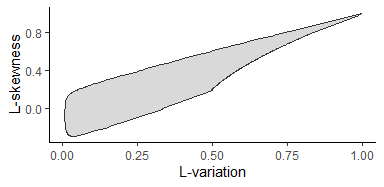Theoretical L-space of BrIII Distribution ## 2.8 Theoretical L-space of BrXII distribution The BrXII Distributions also has two shape parameters and can be presented on the L-moment ratio diagram as a theoretical L-space. LMoFit developed the theoretical L-space of the BrXII distribution and embedded its results as a ggplot in the package data. Users can call the L-space plot of the BrXII as lspace_BrXII and apply any desired adjustments to it as for regular ggplots. lspace_BrXII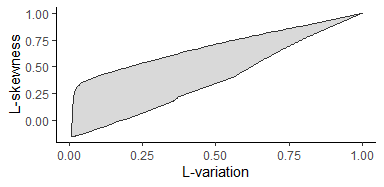Theoretical L-space of BrXII Distribution ## 2.9 Theoretical L-space of GG distribution The GG Distributions is another case of distributions having two shape parameters so it can also be presented on the L-moment ratio diagram with a theoretical L-space. LMoFit developed the theoretical L-space of the GG distribution and embedded its results as a ggplot in the package data. Users can call the L-space plot of the GG as lspace_GG and apply any desired adjustments to it as for regular ggplots. lspace_GG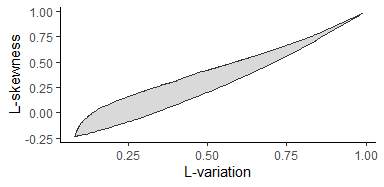Theoretical L-space of GG Distribution ## 2.10 Goodness of fitting on L-moment ratio diagrams There are numerous criteria for comparing the goodness of fitting of probability distributions. A very important preliminary test should be a visual inspection of the L-moment ratio diagrams. The sample, that the distributions are fitted to, is presented on the L-moment ratio diagram as an L-point. If this L-point lies outside the L-space of any two-shape parametric distribution, then the distribution is not valid to describe the sample. For a single sample such as the sample provided as FLOW_AMAX, the test can be implemented by calling the function com_sam_lspace() accounting for ‘compare a sample with an L-space’. This function is valid for the two-shape parametric distributions that are BrIII, BrXII, and GG. The L-point of the sample is presented by a red point mark on the diagrams. com_sam_lspace(sample = FLOW_AMAX, type = "s", Dist = "BrIII")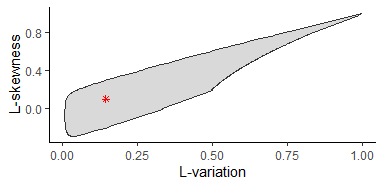Example of single L-points on the L-space of BrIII Distribution com_sam_lspace(sample = FLOW_AMAX, type = "s", Dist = "BrXII")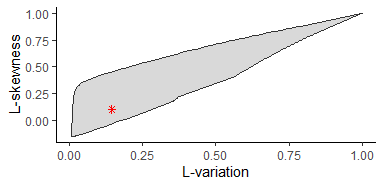Example of single L-points on the L-space of BrXII Distribution com_sam_lspace(sample = FLOW_AMAX, type = "s", Dist = "GG")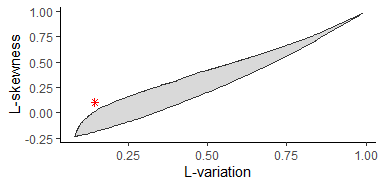Example of single L-points on the L-space of GG Distribution For multiple samples, such as streamflow observed at various sites, the sample of each site is presented by one L-point on the L-moment ratio diagram. Therefore, by implementing the visual test to multiple samples we can identify distributions that have their L-spaces overlaying the greatest portion of L-points and therefore we can select the best regionally valid distribution. com_sam_lspace is developed to be flexible and user friendly. The user can use the same function with multiple sites by changing the type condition from type = "s" to type = "m". Ten hypothetical samples are developed at 10 hypothetical sites and embedded in the package data as FLOW_AMAX_MULT. FLOW_AMAX_MULT is a dataframe with 10 columns where each column describes the sample at one site. colnames(FLOW_AMAX_MULT) <- paste0("site.", 1:10) knitr::kable(head(FLOW_AMAX_MULT), caption = "The first few observations of streamflow at 10 sites") The first few observations of streamflow at 10 sites site.1 site.2 site.3 site.4 site.5 site.6 site.7 site.8 site.9 site.10 589 76 69 317 411 300 158 359 101 258 513 379 244 102 275 357 176 333 18 78 306 57 212 115 103 266 174 146 301 79 394 376 300 189 334 351 19 130 131 141 428 253 325 68 270 140 29 144 170 158 524 39 298 260 222 110 391 50 205 101 An example of a regional visual test is implemented as com_sam_lspace(sample = FLOW_AMAX_MULT, type = "m", Dist = "BrIII", shape = 20)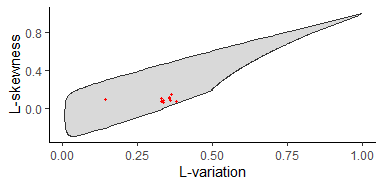Example of multiple L-points on the L-space of BrIII Distribution com_sam_lspace(sample = FLOW_AMAX_MULT, type = "m", Dist = "BrXII", shape = 20)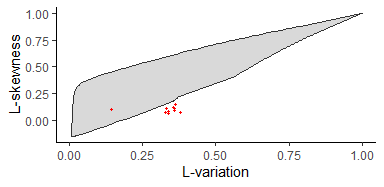Example of multiple L-points on the L-space of BrXII Distribution com_sam_lspace(sample = FLOW_AMAX_MULT, type = "m", Dist = "GG", shape = 20)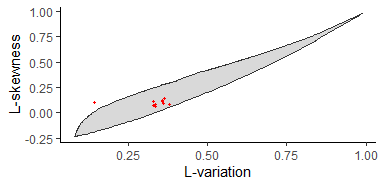Example of multiple L-points on the L-space of GG Distribution These diagrams are still in ggplot format and can be further adjusted by the user. In the last example, the visual test with multiple samples FLOW_AMAX_MULT reveals that the L-space of BrIII combines the greatest amount of L-points and therefore BrIII should be a better candidate compared to BrXII and GG distributions. ## 2.11 Condition of sample L-points as inside/outside of L-spaces As the number of multiple points increases, the corresponding number of L-points increases, and it gets harder to make a visual judgment. For that reason, LMoFit provides an extra function to test the condition of each L-point of the multiple samples and identify it as inside or outside the L-space of interest. All L-points that are overlaid by the L-space are assigned a flag ‘lpoint_inside_lspace’, or ‘lpoint_outside_lspace’ otherwise. flags_BrIII <- con_sam_lspace(sample = FLOW_AMAX_MULT, type = "m", Dist = "BrIII") knitr::kable(head(flags_BrIII), caption = "Flags obtained for BrIII's L-space") Flags obtained for BrIII’s L-space sites condition site.1 lpoint_inside_lspace site.2 lpoint_inside_lspace site.3 lpoint_inside_lspace site.4 lpoint_inside_lspace site.5 lpoint_inside_lspace site.6 lpoint_inside_lspace flags_GG <- con_sam_lspace(sample = FLOW_AMAX_MULT, type = "m", Dist = "GG") knitr::kable(head(flags_GG), caption = "Flags obtained for GG's L-space") Flags obtained for GG’s L-space sites condition site.1 lpoint_outside_lspace site.2 lpoint_inside_lspace site.3 lpoint_inside_lspace site.4 lpoint_inside_lspace site.5 lpoint_inside_lspace site.6 lpoint_inside_lspace By counting the number of times the flag was lpoint_inside_lspace we can exactly identify the number of L-points inside each L-space as counter_BrIII <- nrow(flags_BrIII[flags_BrIII$condition == "lpoint_inside_lspace",])
paste0("the number of L-points inside the L-space of BrIII = ", counter_BrIII)
#>  "the number of L-points inside the L-space of BrIII = 10"
counter_GG <- nrow(flags_GG[flags_GG$condition == "lpoint_inside_lspace",]) paste0("the number of L-points inside the L-space of GG = ", counter_GG) #>  "the number of L-points inside the L-space of GG = 9" Accordingly, we should use BrIII rather than GG as a regional distribution in the latter example. Note: con_samlmom_lspace() is similar to con_sam_lspace(), but it needs the sample L-moments rather than the sample itself and is only applicable to single samples. The sample L-moments are estimated by using get_sample_lmom(). # 3 Step by step guide to frequency analysis Frequency analysis can be implemented by LMoFit in three steps: 1. Estimate sample L-moments using ‘get_sample_lmom()’. 2. Fit a specific distribution, for example, BrIII, to the estimated sample L-moments, using ‘fit_BrIII()’. 3. Estimate probabilities “pBrIII()”, quantiles “qBrIII()”, densities “dBrIII()”, or even return periods “tBrIII()”. The three steps are further explained by an example of fitting BrIII to the embedded sample FLOW_AMAX. Since BrIII distribution is to be used, we will check its validity to describe the sample. con_sam_lspace(sample = FLOW_AMAX, type = "s", Dist = "BrIII") #>  "lpoint_inside_lspace" BrIII passes the visual test since the L-point of the sample is located inside the L-space of BrIII distribution. com_sam_lspace() can also be used for the same purpose. Next, the sample L-moments can be estimated as follows. # Step 1 sample <- FLOW_AMAX samlmoms <- get_sample_lmom(x = sample) After that, the desired distribution can be fitted to the sample L-moments to determine its fitted parameters. # Step 2 parameters <- fit_BrIII(sl1 = samlmoms$sl1, st2 = samlmoms$st2, st3 = samlmoms$st3)

Once the fitted parameters are obtained, frequency analysis can be conducted in different forms to estimate probabilities, quantiles, densities, and return periods.

# Step 3
quantile <- qBrIII(RP = c(5, 10, 25, 50, 100),
para = c(parameters$scale, parameters$shape1, parameters$shape2)) prob <- pBrIII(x = quantile, para = c(parameters$scale, parameters$shape1, parameters$shape2))
dens <- dBrIII(x = quantile,
para = c(parameters$scale, parameters$shape1, parameters$shape2)) T_yrs <- tBrIII(x = quantile, para = c(parameters$scale, parameters$shape1, parameters$shape2))

The results of this example are concluded in the table below.

output <- cbind(Q = round(quantile, digits = 0),
CDF = round(prob, digits = 4),
PDF = round(dens, digits = 5),
T_yrs)
knitr::kable(output, caption = "Example of fitting BrIII distribution to FLOW_AMAX")
Example of fitting BrIII distribution to FLOW_AMAX
Q CDF PDF T_yrs
517 0.80 0.00228 5
576 0.90 0.00117 10
656 0.96 0.00044 25
721 0.98 0.00021 50
791 0.99 0.00010 100

# 4 Acknowledgement

This research is financially supported by the Integrated Modeling Program for Canada (IMPC) project under the umbrella of the Global Water Futures (GWF) program at the University of Saskatchewan, Canada. S.M.P. acknowledges the support of the Natural Sciences and Engineering Research Council of Canada (NSERC Discovery Grant: RGPIN ‐2019 ‐06894 ). In addition, M.Z. acknowledges the Department of Civil, Geological, and Environmental Engineering for the financial support of research through the Departmental Devolved Scholarship.

# 5 References

• Hosking, J.R.M. (2019). L-Moments. R package, version 2.8. link
• Hosking, J.R.M. (1990). L-Moments: Analysis and Estimation of Distributions using Linear Combinations of Order Statistics. Journal of the Royal Statistical Society. Series B 52, 105–124. link
• Papalexiou, S.M., Koutsoyiannis, D. (2016). A global survey on the seasonal variation of the marginal distribution of daily precipitation. Advances in Water Resources 94, 131–145. link
• Zaghloul, M., Papalexiou, S.M., Elshorbagy, A., Coulibaly, P. (2020). Revisiting flood peak distributions: A pan-Canadian investigation. Advances in Water Resources 145, 103720. link